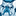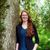# New to Qlik Sense

If you’re new to Qlik Sense, start with this Discussion Board and get up-to-speed quickly.

Announcements
QlikWorld Online 2021, May 10-12: Our Free, Virtual, Global Event REGISTER TODAY
cancel
Showing results for
Search instead for
Did you mean:Creator

## Set Analysis

Hi - I have tried below 2 expressions.

1.sum(Aggr(Sum({<[Period]= {'P3'}>}([Area]/[Total Area])*Time), Key))

2.sum(Aggr(Sum({1<[Period]= {'P3'}>}([Area]/[Total Area])*Time), Key))

I want to lock the value to P3 meaning any period is selected from the list I dont want the value to change but as of now if I select other periods value goes to 0.

Any thoughts please ?

Labels (4)

• ### swuel

1 Solution

Accepted SolutionsMVP

You probably need to put the set analysis in both of the sum() functions:

sum({<[Period]= {'P3'}>}Aggr(Sum({<[Period]= {'P3'}>}([Area]/[Total Area])*Time), Key))

5 RepliesMVP

You probably need to put the set analysis in both of the sum() functions:

sum({<[Period]= {'P3'}>}Aggr(Sum({<[Period]= {'P3'}>}([Area]/[Total Area])*Time), Key))Creator
Author

Its not working...out put is 0.MVP

Can you post a sample file?  Can't tell what's happening without seeing the document...Creator II

Are you selecting from the field "Period" or another field that also represents the period?  If the latter you'll need to exclude it in your set analysis ie OtherField=

What happens with the inner Sum - does it evaluate properly when selecting the period?

Is your data model and data complete such that selecting a period links to all the data fields and nothing is missing?  For example if there is no Time for your selected period then the expression will return 0.Creator
Author
I had the wrong field.This worked.Thank you much.Tags